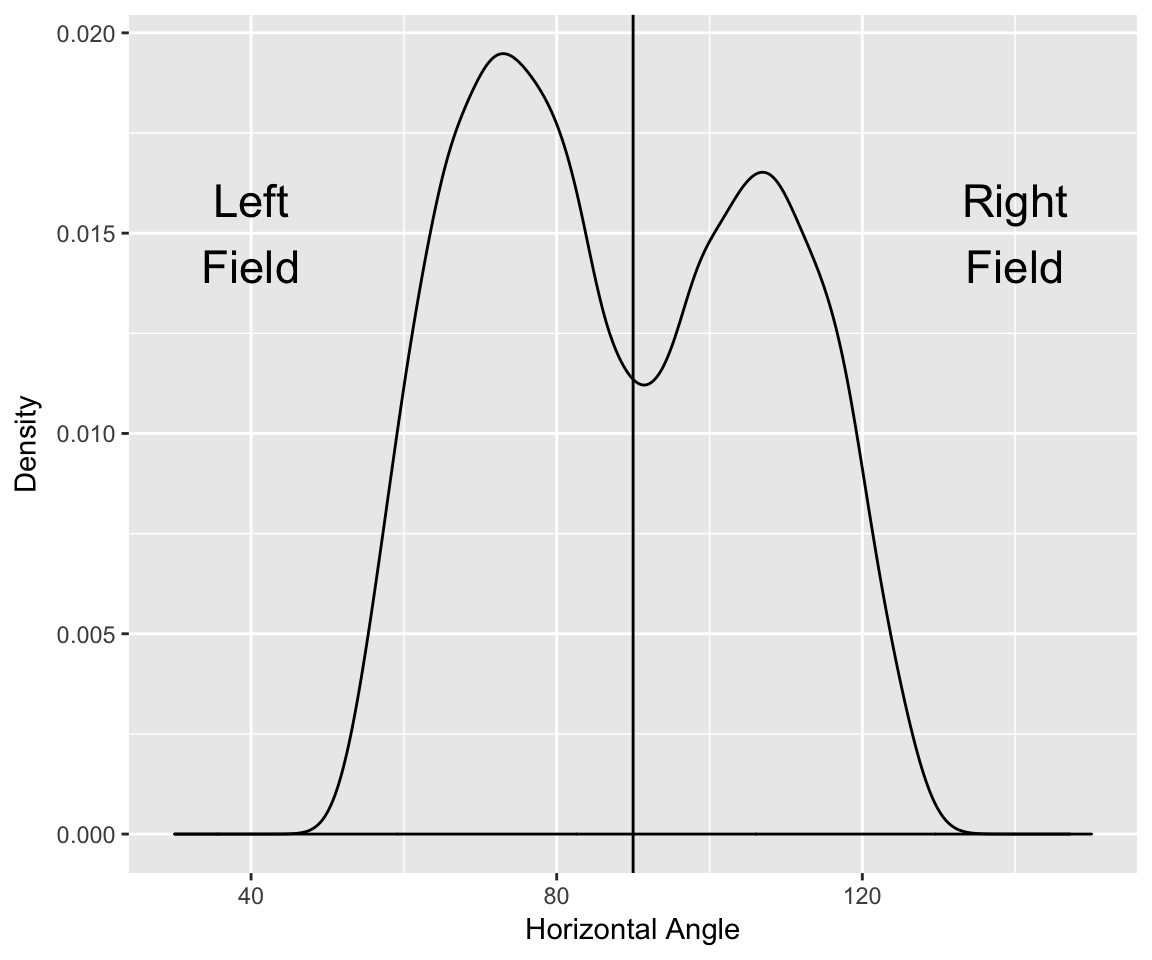### Ballpark Effects

``````library(dplyr)
library(ggplot2)
library(stringr)
library(Lahman)

#### The Data

The ESPN home run tracker http://www.hittrackeronline.com/ contains a number of variables for each home run hit during the current season. I collected this data for five baseball seasons (2012 through 2016) and the csv file homeruns.csv contains data on 24,299 home runs hit during these five seasons.

``````d <- read_csv("https://bayesball.github.io/VB/data/homeruns.csv")
``````## # A tibble: 6 x 16
##      Date Video  Path          Hitter H_Team            Pitcher P_Team
##     <chr> <chr> <chr>           <chr>  <chr>              <chr>  <chr>
## 1 10/3/12 Video  View  Longoria, Evan     TB      Arrieta, Jake    BAL
## 2 10/3/12 Video  View    Johnson, Dan    CHW        Huff, David    CLE
## 3 10/3/12 Video  View Maybin, Cameron     SD  Kintzler, Brandon    MIL
## 4 10/3/12 Video  View  Cano, Robinson    NYY Mortensen, Clayton    BOS
## 5 10/3/12 Video  View    Moore, Tyler    WSH         Lee, Cliff    PHI
## 6 10/3/12 Video  View  Longoria, Evan     TB     Tillman, Chris    BAL
## # ... with 9 more variables: Inning <int>, Ballpark <chr>,
## #   `Type/Luck` <chr>, True_Dist <int>, Speed_off_Bat <dbl>,
## #   Elevation_Angle <dbl>, Horiz_Angle <dbl>, Apex <int>, N_Parks <int>``````

#### (Figure 6.4) distribution of horizontal angle

In the book, I define the horizontal angle which is 180 - Horiz_Angle where `Horiz_Angle` is the definition of the horizontal angle on the website.

Here is a density plot of the collection of horizontal angles.

``````ggplot(d, aes(180 - Horiz_Angle)) +
geom_density() +
xlim(30, 180 - 30) +
xlab("Horizontal Angle") +
ylab("Density") +
geom_vline(xintercept=90) +
annotate("text", x=40, y=0.015,
label="Left\nField", size=6) +
annotate("text", x=140, y=0.015,
label="Right\nField", size=6)``````#### (Figure 6.5) relationship of distance and horizontal angle

Here I graph the horizontal angle against the home run distance and add a smoothing curve to show the general pattern.

``````ggplot(d, aes(180 - Horiz_Angle, True_Dist)) +
geom_point(alpha=0.1) + geom_smooth() +
ylim(300, 500) + xlim(45, 130) +
xlab("Horizontal Angle") +
ylab("Distance")``````